## word2vec （CBOW、分层softmax、负采样）

1. wordvec的概念
2. 语言模型训练的两种模型CBOW+skip gram
3. word2vec 优化的两种方法：层次softmax+负采样
4. gensim word2vec默认用的模型和方法

## 1. 词表示：

• 词的独热表示：onehot （词之间是孤立的）
• onehot:
• 思想：假设词表大小为N， 则每个单字表示为N维向量； 每个单字只有1位为1，其他为0；茫茫0海中一个1
• 缺点：词之间是孤立的；维度太大
• 词的分布式表示：（能描述词之间的语义关系）
• 基于矩阵的分布式表示
• 基于聚类的分布式表示
• 基于神经网络的分布表示，词嵌入 word embedding
• 将01表示改为浮点数表述；降维
• word2vec是用神经网络训练语言模型（NNLM）过程中得到的参数.
• 其他概念
• 语言模型： 就是一段文字成为句子的概率； 经常用的ngram, n=2或3

## 2. NNLM 神经网络语言模型

### 2.1 CBOW 连续词袋模型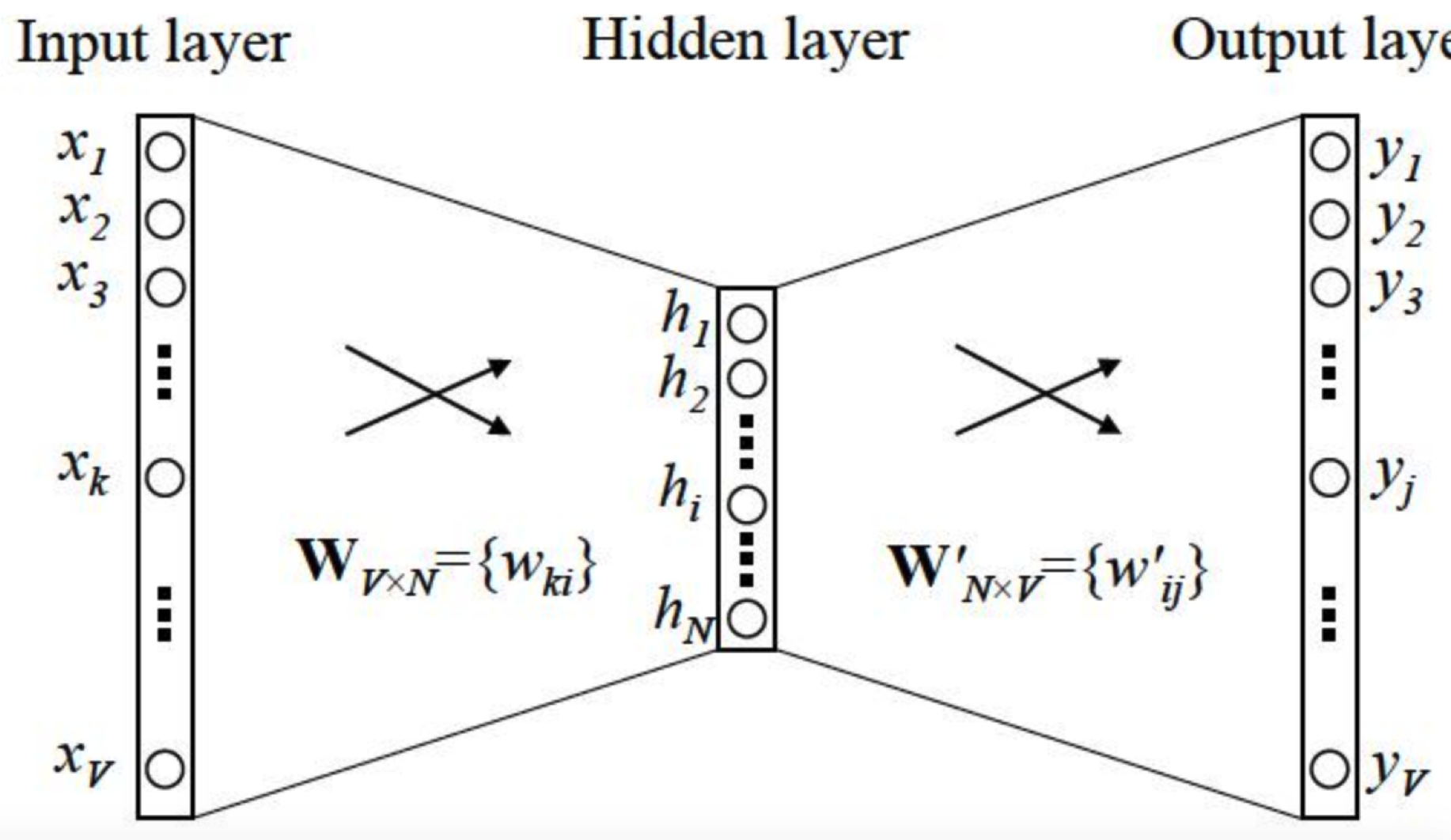• 变量：词表大小V, 要嵌入到N维空间中，C/2是窗口大小，即上下文取几个term(不算当前term)；- N一般取50~300
• 步骤：参考自 https://www.zhihu.com/question/44832436
• input layer：窗口内的C个词的onehot表示
• input layer -> hidden layer：（V维表示降到N维表示）
• 通过权重矩阵$$W_{V\times N}$$ ,将 $$V \times C$$映射为 $N \times C$
• hidden layer + 激活函数：C个词表示为1个词
• word2vec中激活函数，用了简单取平均；
• hidden layer -> output layer：1个N维词还原到高维V维中；
• 通过权重矩阵$$W_{N\times V}^{'}$$ ,将 $$N \times 1$$映射为 $$V\times 1$$
• output layer + softmax 激活函数，将值归一化到0~1之间 y
• 用BP+梯度下降优化cost function y和真实y之间的距离，迭代优化参数 $$W、W^{'}$$
• 收敛result：y是V维向量，每个元素取值0~1, 将最大元素值，还原为onehot编码，就是最终结果了。

## 3. word2vec用的优化方法

word2vec结合了CBOW, skip-gram的方法 训练得到参数$$W$$, 但在计算中做了很多优化；

1. 词表维度大；
2. softmax计算量大；

### 3.1 hierarchical softmax 层次softmax

/ˌhī(ə)ˈrärkikəl/

• huffman: 带权路径长度最短为目标，可以用log(V)长度的编码表示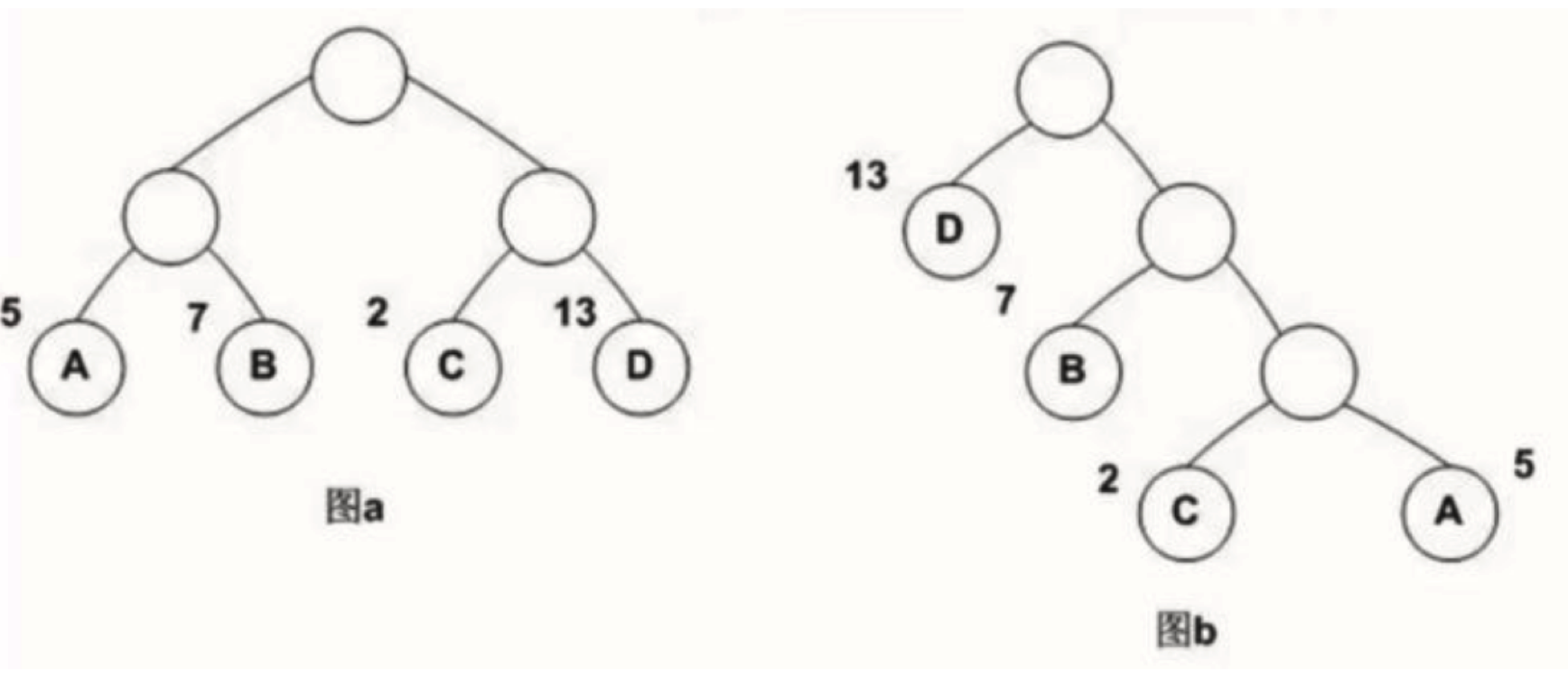带权路径长度WPL：
• 层次softmax
softmax需要对每个词语都计算输出概率，并进行归一化，计算量很大；
进行softmax的目的是多分类，那么是否可以转成多个二分类问题呢， 如SVM思想？ 从而引入了层次softmax

• 示意图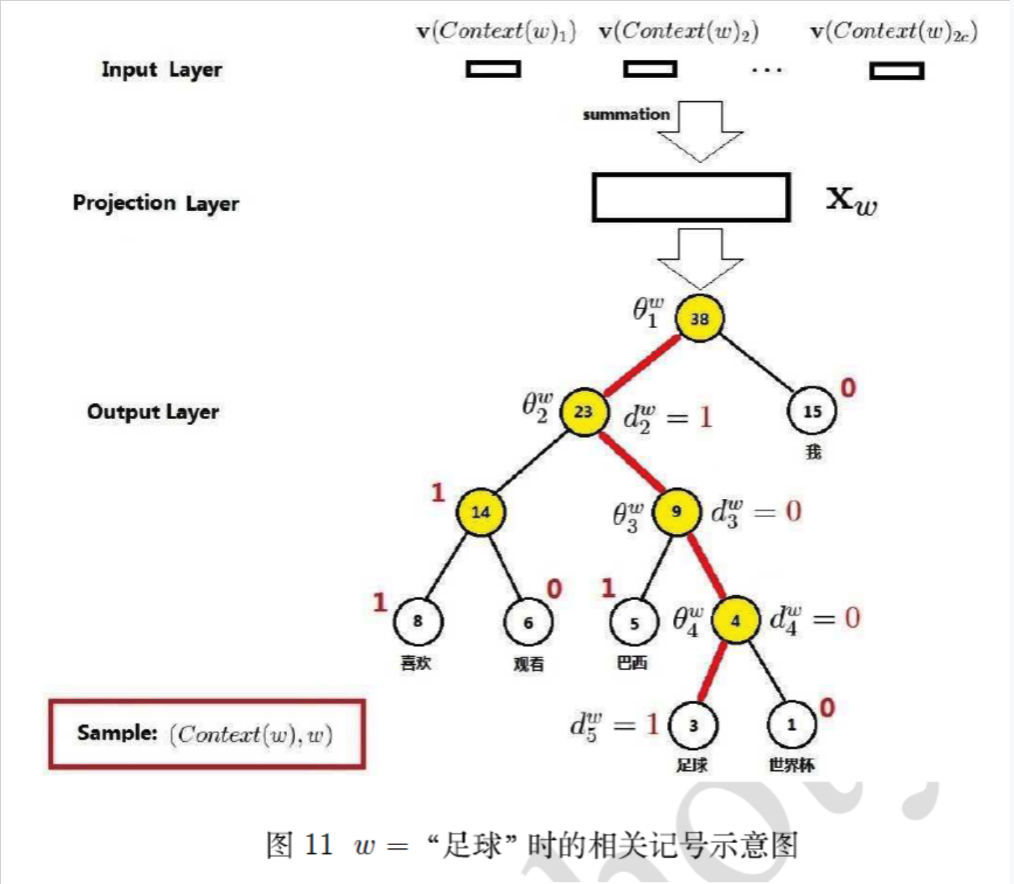• 为什么有效？
• 1）用huffman编码做词表示
• 2）把N分类变成了log(N)个2分类。 如要预测的term（足球）的编码长度为4，则可以把预测为'足球'，转换为4次二分类问题，在每个二分类上用二元逻辑回归的方法（sigmoid）；
• 3）逻辑回归的二分类中，sigmoid函数导数有很好的性质，$$\sigma^{'}(x) = \sigma(x)(1-\sigma(x))$$
• 4）采用随机梯度上升求解二分类，每计算一个样本更新一次误差函数
• 参考自：http://flyrie.top/2018/10/31/Word2vec_Hierarchical_Softmax/
• gensim的word2vec 默认已经不采用分层softmax了, 因为$log_2 1000=10$也挺大的；如果huffman的根是生僻字，则分类次数更多；

### 3.2 高频词抽样+负采样

1. 将常见的单词组合（word pairs）或者词组作为单个“words”来处理
2. 对高频次单词进行抽样来减少训练样本的个数
3. 对优化目标采用“negative sampling”方法，这样每个训练样本的训练只会更新一小部分的模型权重，从而降低计算负担

#### 3.2.1 对高频词抽样

•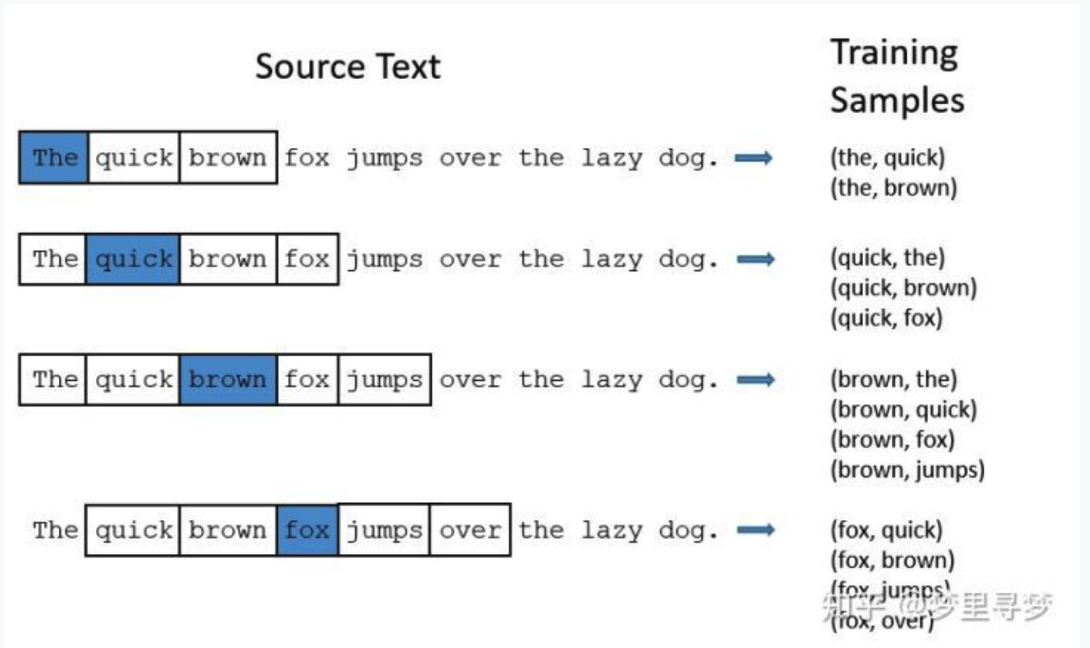• 抽样率 $$P(w_{i}) = (\sqrt{\frac{Z(w_i)}{t}}+1)\times \frac{t}{Z(w_i)} = \sqrt{\frac{t}{Z(w_i)}} + \frac{t}{Z(w_i)}$$ ，其中
• $$Z(w_i)$$是词在语料中的出现概率，反比关系，越高频词抽的越少；
• $$t$$是设定的阈值，正比关系，$$t$$越大，不同频率单词的采样概率差异越大； gensim word2vec中默认值是0.001
• 抽样率直观理解
•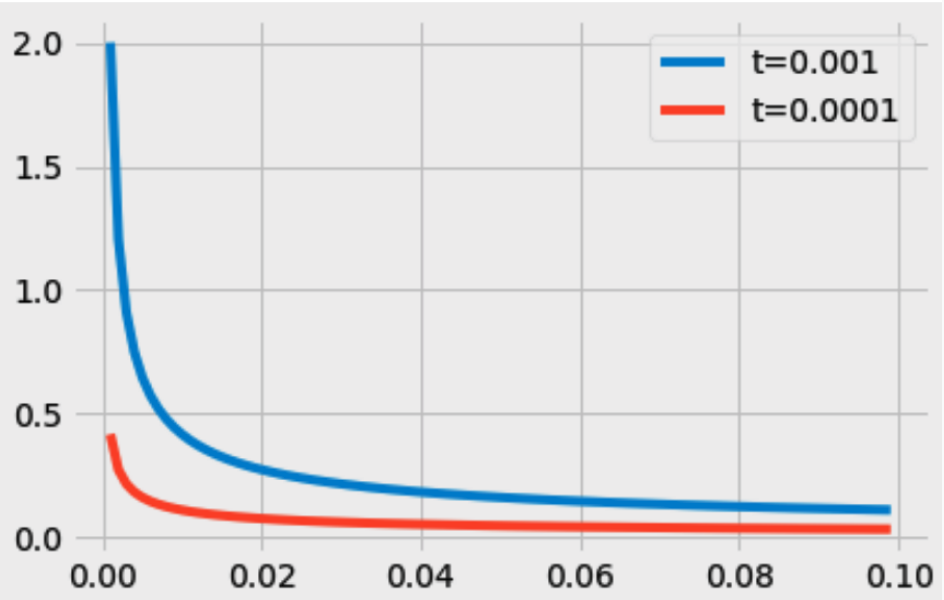import numpy as np
import matplotlib.pyplot as plt

x = np.array(range(1, 100)) / 1000.0
t1 = 0.001
t2 = 0.0001
y1 = np.power(t1/x, 0.5) + t1/x
y2 = np.power(t2/x, 0.5) + t2/x
plt.plot(x, y1, '-', label='t=0.001')
plt.plot(x, y2, '-', label='t=0.0001')
plt.legend()

#### 3.2.2 负采样 Negative sampling

• 问题：在NNLM中对于每个样本w, 都要更新一次hidden->output的参数阵$$W^{'}_{N\times V}$$，计算量很大；比如词表大小V=10000, N=300, 那么权重矩阵有300万个参数；
• 思想：如果每次只更新目标词（正例 positive word）和少数几个负例（negative words）, 那么计算量会显著减少； 比如如果我们每次只更新M=1+5个，则更新了6*300=1800, 1800/300万=0.06%； 计算量减少了3个数量级。
• negative words如何选择：根据词表中出现的概率，出现概率越高，被选作负样本的概率越高；
• $$P(w_i) = \frac{f(w_i)^{3/4}}{\sum_{j=0}^{v}{f(w_j)^{3/4}}}$$, 其中$$f(w_j)$$是出现的概率，3/4是经验值，比线性关系更弱化了些；

## 4. gensim word2vec默认参数（CBOW+负采样）

from gensim.models import Word2Vec
word2vec.Word2Vec(sentences=None, size=100, alpha=0.025, window=5, min_count=5, max_vocab_size=None, sample=0.001, seed=1, workers=3, min_alpha=0.0001, sg=0, hs=0, negative=5, ns_exponent=0.75, cbow_mean=1,  iter=5, null_word=0, trim_rule=None, sorted_vocab=1, batch_words=10000, compute_loss=False, callbacks=(), max_final_vocab=None)
• 上下文窗口大小：window=5
• 忽略低频次term：min_count=5
• 语言模型是用CBOW还是skip-gram？sg=0 是CBOW
• 优化方法是用层次softmax还是负采样：hs=0 是负采样
• 负采样样本数: negative=5 (一般设为5-20)
• 负采样采样概率的平滑指数：ns_exponent=0.75
• 高频词抽样的阈值 sample=0.001

0条评论